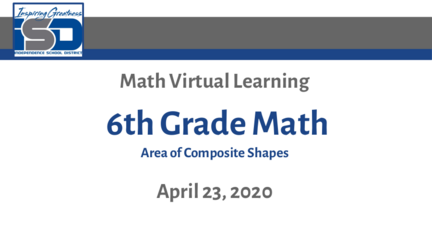# Finding the Area of a Triangle and Other Composite ShapesContributed by:This pdf help us to find us the area of triangle and other composite Shapes that consists of different polygons like triangle, square and parrllelogram
1. Math Virtual Learning
Area of Composite Shapes
April 23, 2020
Lesson: April 23, 2020
Objective/Learning Target:
Students will find the area of composite shapes/polygons by
composing or decomposing the shapes into rectangles and
triangles.
3. Let’s Get Started!
Watch This Video:
4. Bell Ringer:
b=2
b=4
h=4
h=7
A = (2 x 4) / 2
A=4x7
= 4 sq. un.
= 28 sq. un.
b = 2.1
b = 21
h = 1.8
h = 12
A = (2.1 x 1.8) / 2
A = 21 x 12
= 1.89 sq. un.
= 252 sq. un.
6. There are many other ways
to divide trapezoids PQRS.
Dividing the trapezoid into
rectangles and triangles is called
decomposing.
After decomposing the trapezoid
PQRS into triangles and
rectangles, you can find the area
of the smaller pieces using the
Finally, add the smaller areas to
find the total area of the trapezoid
PQRS.
7. To find the side
length of a square,
find the square Putting it all
root of the area. together….
8. Find the area of each composite figure below.
Triangle: Rectangle: Triangle:
b= 8, h= 19 - 13 = 6 A = l x w = 11 x 4 = 44 sq. cm b = 7, h = 8
A = (8 x 6)/2 = 48/2 = 24 sq. in A = (b x h)/2 = (7 x 8)/2
Square: = 56/2 = 28 sq. yd.
Rectangle: A = l x w = 5 x 5 = 25 sq. cm
A= l x w = 13 x 8 = 104 sq. in Square:
Combined Area: A = l x w = 8 x 8 = 64 sq. yd.
Combined Area: A = 44 + 25 = 69 sq. cm
A= 24 + 104 = 128 sq. in Combined Area:
A = 28 + 64= 92 sq. yd.
10. Complete the triangle-square-circle reflection for today’s lesson.
What were the What squared What questions
three main (made sense) do you still have
points of today’s with you from circling around